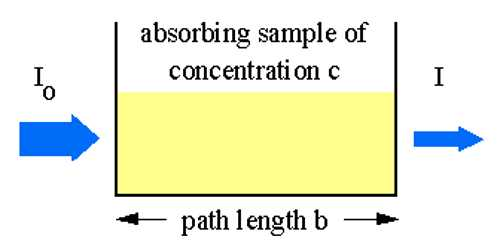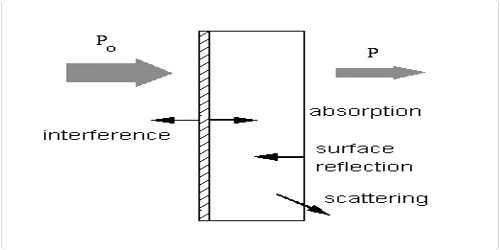Chemistry

# Absorption of Light: Beer-Lambert LawAbsorption of Light: Beer-Lambert Law

If material bodies are exposed to radiation, part of the incident radiation is absorbed, a part is scattered and a part is transmitted. As a result of absorption the intensity of light passing through material bodies, i.e., the intensity of transmitted light, decreases. The fraction of incident light absorbed depends on the thickness of the absorbing medium. Lambert derived a qualitative relationship between the decreases in intensity of a monochromatic light due to the passage through a homogeneous medium of thickness dx and the intensity of light l. This law is known as Lambert’s law, and may be stated as-

The decrease in intensity of light with thickness of the absorbing medium at any point is directly proportional to the intensity of light.

The proportion of the light absorbed will depend on how many molecules it interacts with. Suppose you have got a strongly coloured organic dye. If it is in a reasonably concentrated solution, it will have a very high absorbance because there are lots of molecules to interact with the light. However, in an incredibly dilute solution, it may be very difficult to see that it is colored at all. The absorbance is going to be very low.Mathematically it can be expressed as,

– (dl/dx) ∞ l … … … (1)

where dl is a small decrease in intensity of light upon passing through a small distance dx and l is the intensity of the monochromatic light just before entering the medium. Equation (1) may be written as,

– (dl/ds) = al … … … (2)

where (dl/ds) is the rate of decrease of intensity with thickness dx, a is called the absorption co-efficient. Integration of equation (2) after rearrangement gives,

– ln l = ax + C

Where C is a constant of integration.